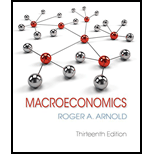# What is the effect on equilibrium price and quantity of the following? a. A decrease in demand that is greater than the increase in supply b. An increase in supply c. A decrease in supply that is greater than the increase in demand d. A decrease in demand### Macroeconomics

13th Edition
Roger A. Arnold
Publisher: Cengage Learning
ISBN: 9781337617390

#### Solutions

Chapter
Section### Macroeconomics

13th Edition
Roger A. Arnold
Publisher: Cengage Learning
ISBN: 9781337617390
Chapter 3.3, Problem 3ST
Textbook Problem
1 views

## What is the effect on equilibrium price and quantity of the following?a. A decrease in demand that is greater than the increase in supplyb. An increase in supplyc. A decrease in supply that is greater than the increase in demandd. A decrease in demand

(a)

To determine

The effect on equilibrium price and quantity.

### Explanation of Solution

A decrease in demand that is greater than the increase in supply leads to a reduction in equilibrium price and equilibrium quantity...

(b)

To determine

The effect on equilibrium price and quantity.

(c)

To determine

The effect on equilibrium price and quantity.

(d)

To determine

The effect on equilibrium price and quantity.

### Still sussing out bartleby?

Check out a sample textbook solution.

See a sample solution

#### The Solution to Your Study Problems

Bartleby provides explanations to thousands of textbook problems written by our experts, many with advanced degrees!

Get Started